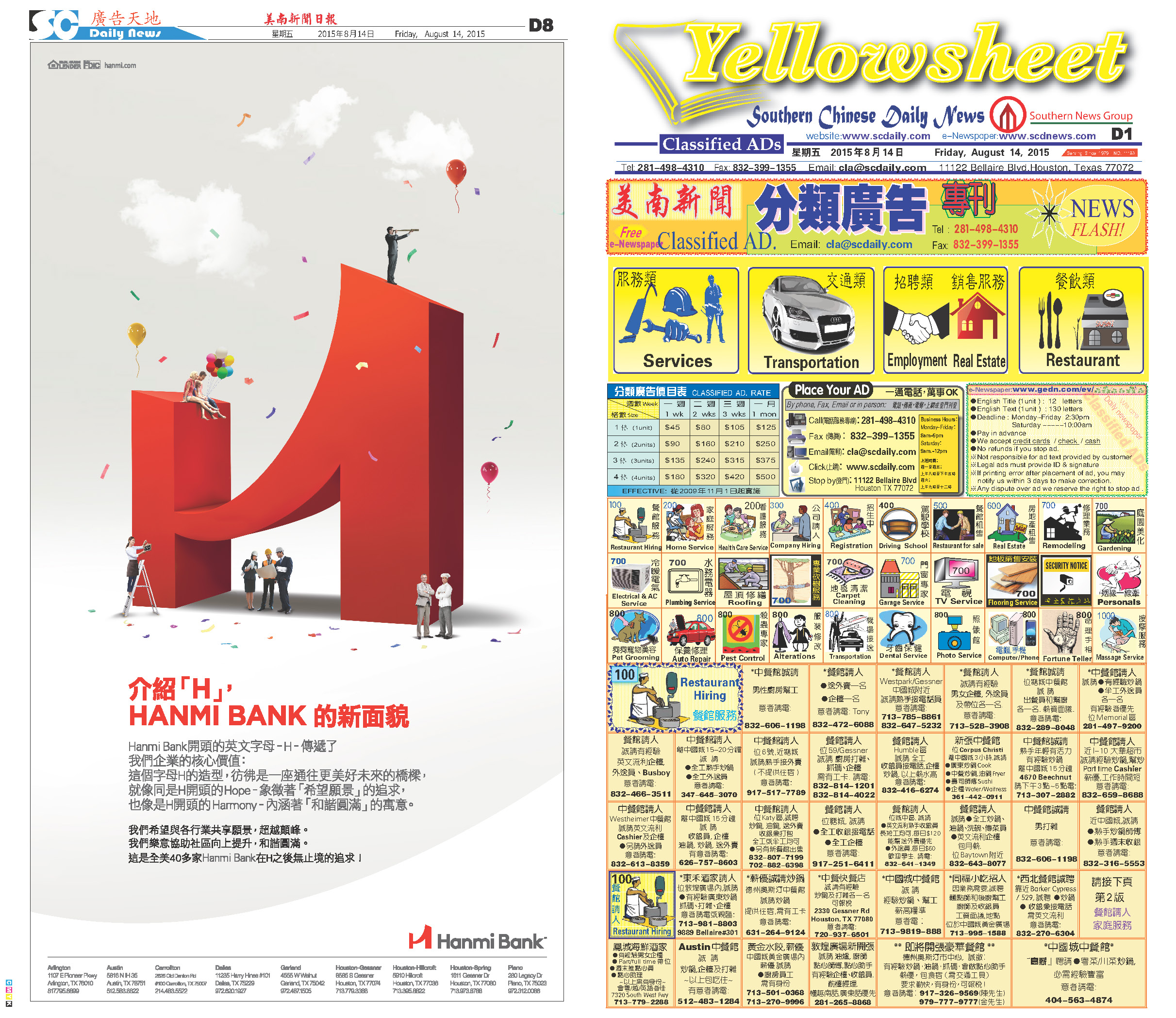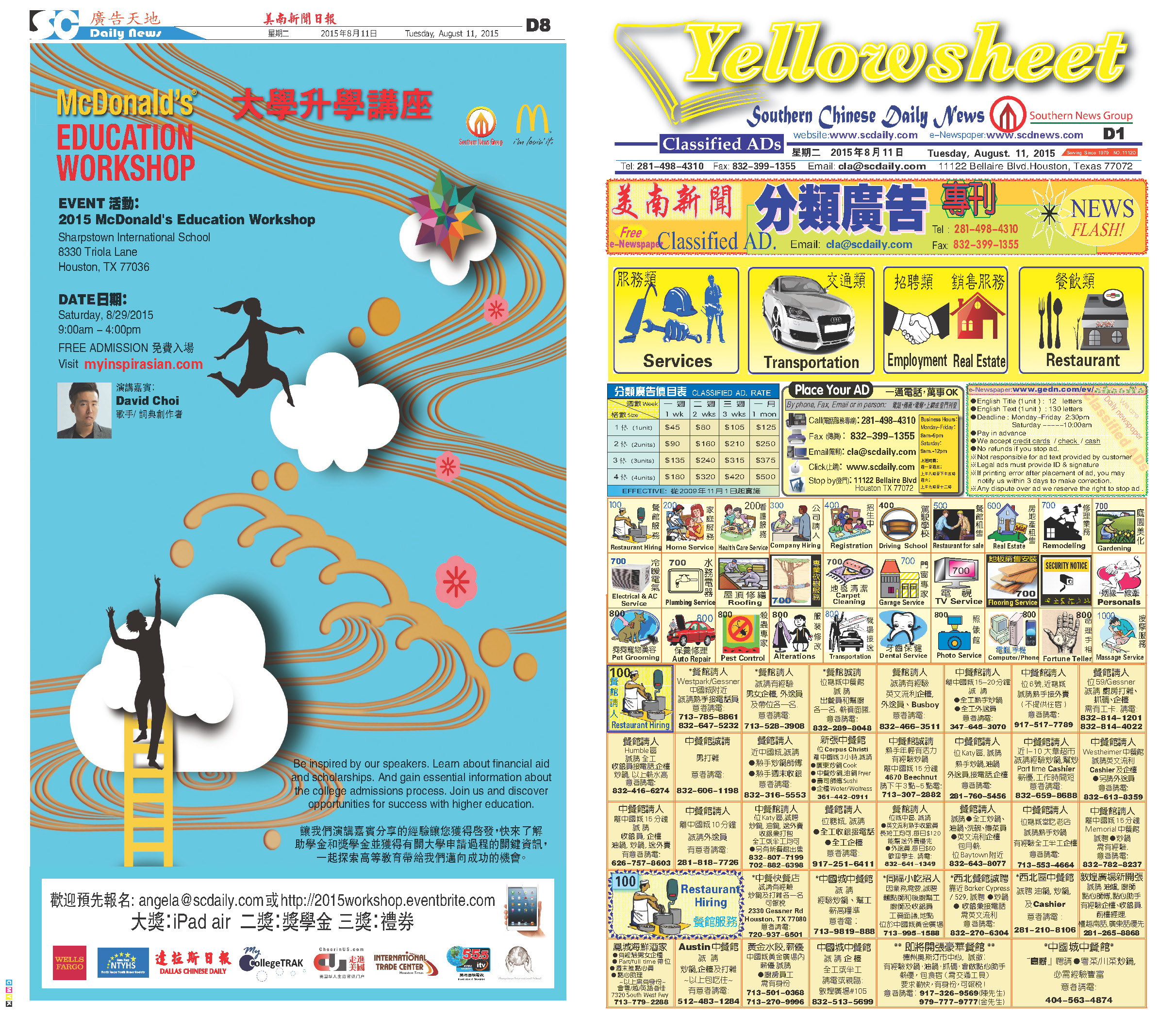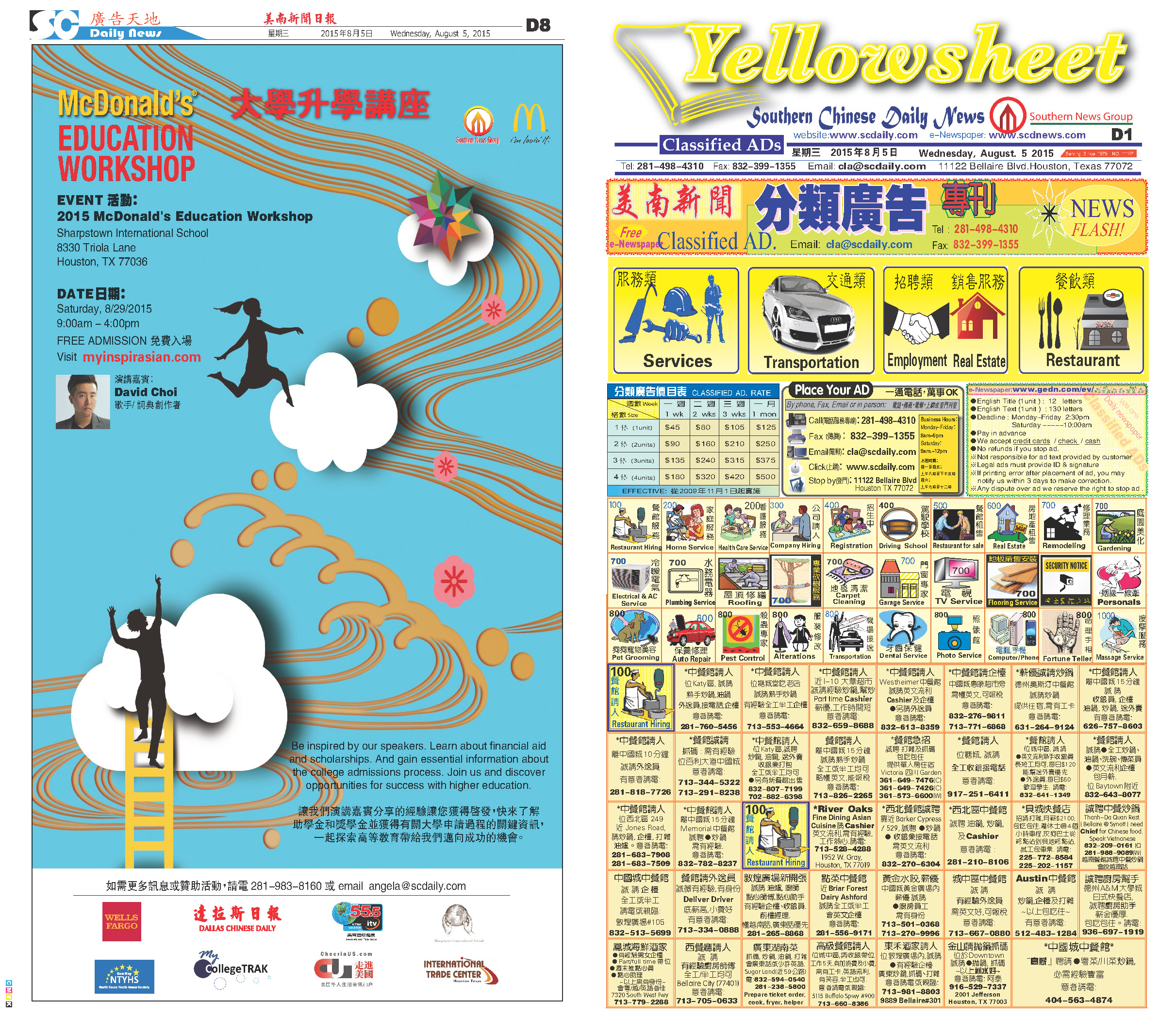150815 Epaper

 A Section B SectionC Section D Section150814 Epaper

 A Section B SectionC Section D Section150813 Epaper

 A Section B SectionC Section D Section150812 Epaper

 A Section B SectionC Section D Section150811 Epaper

 A Section B SectionC Section D Section150810 Epaper

 A Section B SectionC Section D Section150809 Epaper

 A Section B SectionC Section D Section150808 Epaper

 A Section B SectionC Section D Section150806 Epaper

 A Section B SectionC Section D Section150807 Epaper

 A Section B SectionC Section D Section150805 Epaper

 A Section B SectionC Section D Section